GFG App
Open AppBrowser
Continue

# Class 10 RD Sharma Solutions – Chapter 14 Coordinate Geometry – Exercise 14.3 | Set 2

### Question 21. Find the ratio in which the point P(-1, y) lying on the line segment joining A(-3, 10) and B(6, -8) divides it. Also, find the value of y.

Solution:

Assume P divide A(-3, 10) and B(6, -8) in the ratio of k : 1

Given: coordinates of P as (-1, y)

After applying the section formula for x – coordinate,

We will getTherefore,

AB is divided by point P in the ratio of 2 : 7

By applying the value of k, to find the y-coordinate

We will gety = (-16 + 70)/(2 + 7) = 54/9

y = 6

Hence,

The y-coordinate of P is 6.

### Question 22. Find the coordinates of a point A, where AB is the diameter of circle whose center is (2, -3) and B is (1, 4).

Solution:

Assume the coordinates of point A be (x, y)

Given: AB is the diameter,

So the center in the mid-point of the diameter

Thus,

(2, -3) = (x + 1/ 2, y + 4/2)

2 = x + 1/2 and -3 = y + 4/2

4 = x + 1 and -6 = y + 4

x = 3 and y = -10

Hence, the coordinates of A are (3, -10)

### Question 23. If the points (-2, 1), (1, 0), (x, 3), and (1, y) form a parallelogram, find the values of x and y.

Solution:

Consider A(-2, 1), B(1, 0), C(x , 3) and D(1, y) are the given points of the parallelogram.

As we know that the diagonals of a parallelogram bisect each other.

Thus,

Coordinates of mid-point of AC = Coordinates of mid-point of BD

((x – 2)/2, (3 – 1)/2) = (1+1)/2, (y + 0)/2

((x – 2)/2, 1) = (1, y/2)

(x – 2)/2 = 1

x – 2 = 2

x = 4

and y/2 = 1

y = 2

Hence, the value of x is 4 and the value of y is 2.

### Question 24. The points A(2, 0), B(9, 1), C(11, 6), and D(4, 4) are the vertices of a quadrilateral ABCD. Determine whether ABCD is a rhombus or not.

Solution:

Given: A(2, 0), B(9, 1), C(11, 6) and D(4, 4).

Mid-point of AC coordinates areMid-point of BD coordinates areHere,

Coordinates of the mid-point of AC ≠ Coordinates of mid-point of BD,

ABCD is not a parallelogram.

Hence,

ABCD cannot be a rhombus too.

### Question 25. In what ratio does the point (-4,6) divide the line segment joining the points A(-6,10) and B(3,-8)?

Solution:

Assume the line segment AB is divided by point (-4, 6) in the ratio of k : 1.

After applying the Section Formula,

We will get-4k -4 = 3k – 6

7k = 2

k : 1 = 2 : 7

We can also check for the y-coordinate also.

Hence,

The ratio in which the line segment AB is divided by point (-4,6) is 2 : 7.

### Question 26. Find the ratio in which the y-axis divides the line segment joining the points (5, -6) and (-1, -4). Also, find the coordinates of the point of division.

Solution:

Assume P(5, -6) and Q(-1, -4) be the given points.

Consider the line segment PQ is divided by y-axis in the ratio k : 1.

After applying the Section Formula for the x-coordinate (as it’s zero)

We will get,-k + 5 = 0

k = 5

Therefore,

The ratio in which the y-axis divides the given 2 points is 5 : 1

Now further, for finding the coordinates of the point of division

On putting k = 5, we will getTherefore,

The coordinates of the point of division are (0, -13/3)

### Question 27. Show that A(-3, 2), B(-5, 5), C(2, -3), and D(4, 4) are the vertices of a rhombus.

Solution: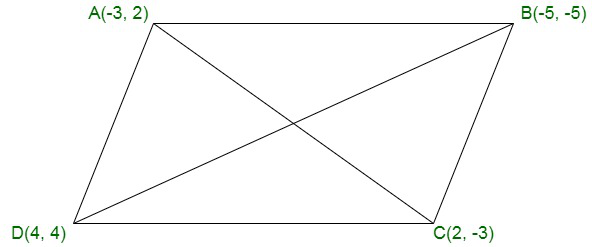Given: A(-3, 2), B(-5, 5), C(2, -3) and D(4, 4)

Further,

Mid-point of AC coordinates areAnd,

Mid-point of BD coordinates areTherefore,

The mid-point for both the diagonals are the same.

Thus,

ABCD is a parallelogram.

Now,

For the sidesAB = BC

We can see that ABCD is a parallelogram with adjacent sides equal.

Therefore,

ABCD is a rhombus.

### Question 28. Find the lengths of the medians of a ΔABC having vertices at A(0, -1), B(2, 1), and C(0, 3).

Solution: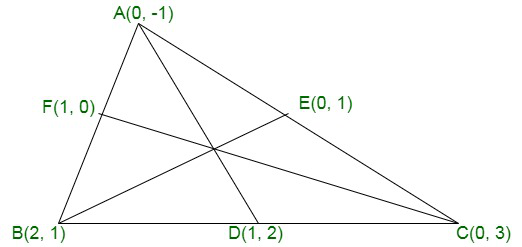Assume AD, BE and CF be the medians of ΔABC

Now,

Coordinates of D are= (1, 2)

Coordinates of E are= (0, 1)

Coordinates of F are= (1, 0)

Further,

The length of the medians

Length of the median AD == √10 units

Length of the median BE == 2 units

Length of the median CF == = √10 units

### Question 29. Find the lengths of the median of a ΔABC having vertices at A(5, 1), B(1, 5), and C(-3, -1).

Solution: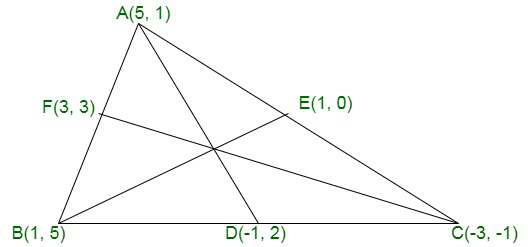Given: Vertices of ΔABC as A(5, 1), B(1, 5) and C(-3, -1).

Consider AD, BE and CF be the medians

Coordinates of D are= (-1, 2)

Coordinates of E are= (1, 0)

Coordinates of F are= (3, 3)

Further,

The length of the medians

Length of the median AD == √37 units

Length of the median BE == 5 units

Length of the median CF == √52 units

### Question 30. Find the coordinates of the point which divide the line segment joining the points (-4, 0) and (0, 6) in four equal parts.

Solution: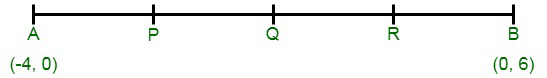Consider A(-4, 0) and B(0, 6) as they are the given points

And,

Assume P, Q and R be the points which divide AB is four equal points, as shown in the fig.

Thus,

As we know that AP : PB = 1 : 3

By applying the Section Formula the coordinates of P areAnd,

We can see that Q is the mid-point of AB

Thus, the coordinates of Q areFinally,

The ratio of AR : BR is 3 : 1

Then, after applying the Section Formula the coordinates of R are### Question 31. Show that the mid-point of the line segment joining the points (5, 7) and (3, 9) is also the mid-point of the line segment joining the points (8, 6) and(0, 10).

Solution:

Assume M be the mid-point of AB. Coordinates of the mid-point of this line segment joining two points A (5, 7) and B (3, 9).Now coordinates of the mid-point of the line segment joining the points (8, 6) and (0, 10) are;Thus, this is the same as the first case.

### Question 32. Find the distance of the point (1, 2) from the mid-point of the line segment joining the points (6, 8) and (2, 4).

Solution:

Assume M be the mid-point of the line segment joining the points (6, 8) and (2, 4)

Now

Coordinates of M will beNow,

Distance between the points (4, 6) and (1, 2)= 5 units

### Question 33. If A and B are (1, 4) and (5, 2) respectively, find the co-ordinates of P WhenSolution:

Here, Point P divides the line segment joining the points (1, 4) and (5, 2) in the ratio of AP : PB = 3 : 4

Coordinates of P will be### Question 34. Show that the points A (1, 0), B (5, 3), C (2, 7), and D (-2, 4) are the vertices of a parallelogram.

Solution:

If ABCD is a parallelogram,

Then its diagonal AC and BD will bisect each other at O

Consider O is the mid-point of AC,

Then coordinates of O will be;And assume O is the mid-point of BD,

Then coordinates of O will be;We see that coordinates of the mid-points of AC and BD are same

Therefore, AC and BD  bisect each other at O

Now, length of ACand length of BD =We can see that AC = BD

Therefore, ABCD is a rectangle.

### Question 35. Determine the ratio in which the point P (m, 6) divides the join of A (-4, 3) and B (2, 8). Also, find the value of m.

Solution:

Assume the ratio be r : s in which P (m, 6) divides the line segment joining the points A (-4, 3) and B (2, 8)

Therefore,and⇒ 8r + 3s = 6r + 6s

⇒ 8r – 6r = 6s – 3s

⇒ 2r = 3sTherefore,

Ratio is 3 : 2

Now,Hence, m = -2/5

### Question 36. Determine the ratio in which the point (-6, a) divides the join of A (-3, -1) and B (-8, 9). Also, find the value of a.

Solution:

Assume the point P (-6, a) divides the join of A (-3, -1) and B (-8, 9) in the ratio m : n

Therefore,-6 = (-8m -3n)/(m + n)

-6m – 6n = -8m – 3n

8m – 6m = 6n – 3n

2m = 3n

m/n = 3/2

Therefore,

Ratio = 3 : 2

and### Question 37. ABCD is a rectangle formed by joining the points A (-1, -1), B (-1, 4), C (5, 4), and D (5, -1). P, Q, R, and S are the mid-points of sides AB, BC, CD, and DA respectively. Is the quadrilateral PQRS a square ? a rectangle ? or a rhombus? Justify your answer.

Solution:

ABCD is a rectangle whose vertices are A (-1,-1), B (-1,4), C (5, 4) and D (5, -1).

P, Q, R, and S are the mid-points of the sides AB, BC, CD and DA respectively

And are joined PR and QS are also joined.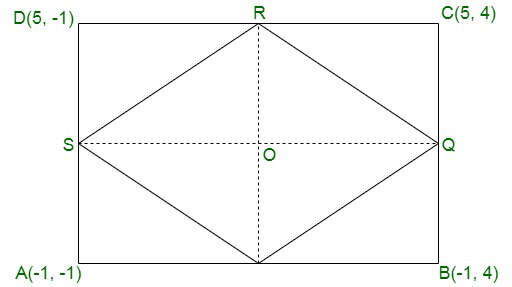Now coordinates of P will beSimilarly, the coordinates of Q, will be:Coordinates of R will be:Coordinates of S will be:Coordinates of P (-1, 3/2), Q (2, 4), R(5, 3/2) and S (2, -1)Now, assume the diagonals PQ and QS intersect each other at O

Assume O is the mid-point of PR,

Then coordinates of O will beSimilarly, of O is the mid-point of QS, then the coordinates of O will beNow, we see that the coordinates of O in both case is same and adjacent sides are also equal

Then it may be a square or a rhombus

Now length of PR =And length of OSBecause diagonal are not equal

Hence, PQRS is a rhombus.

### Question 38. Points P, Q, R, and S divide the line segment joining the points A (1, 2) and B (6, 7) in 5 equal parts. Find the coordinates of the points P, Q, and R.

Solution:

Points P, Q, R and S divides AB in 5 equal parts and assume coordinates of P, Q, R and S are,

(x1, y1), (x2, y2), (x3, y3), (x4, y4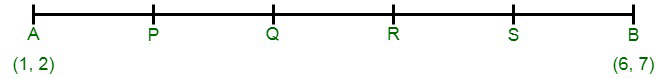⇒ P divides AB in ratio 1 : 4

Therefore,Hence, Coordinates of P are (2, 3)

⇒ Q divides AB in the ratio 2 : 3

Therefore,Hence, Coordinates of 3, 4

⇒ R divides AB in ration 3 : 2

Therefore,Hence, Coordinates of R are (4, 5).

### Question 39. If A and B are two points having coordinates (-2, -2) and (2, -4) respectively, find the coordinates of P such that AP = 3/7 AB

Solution:

AP = 3/7 AB

7AP = 3AB

7AP = 3(AP + BP)

⇒ 7AP = 3AP + 3BP

⇒ 7AP – 3AP = 3BP

⇒ 4 AP = 3 BP

⇒Therefore,

AP : BP = 3 : 4

Because P divides AB in the ratio of 3 : 4 whose end points are A(-2, -2) and B(2, -4)

Therefore, Coordinates of P will beTherefore,

Coordinates of P will be### Question 40. Find the coordinates of the points which divide the line segment joining A (-2, 2) and B (2, 8) into four equal parts.

Solution:

Assume P, Q and R divides the line segment AB in four equal parts

Co-ordinates of A are (-2, 2) and of B are (2, 8)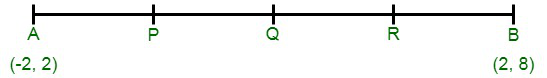It can be seen that Q divides AB in two equal parts while P bisects AQ and R, bisect QB.

Now,

Coordinates of Q will be :Similarly, coordinates of P will be:Coordinates of R will be:Hence, Coordinates of P are(1, 7/2)

Coordinates of Q are (0, 5)

Coordinates of R are (1, 13/2)

My Personal Notes arrow_drop_up